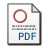# Numerical and Monte Carlo Methods to make Normal Residues in Regression

by Ciuiu, Daniel
Published in Romanian Journal of Economic Forecasting
, 2009, volume 12 issue 4, 119-131Requires a PDF viewer such as Xpdf or Adobe Acrobat Reader  137Kb

## Abstract

The Jarque-Bera normality test  verifies if the residues of the  regression hyper-plane are normal random  variables. In this paper we present some  numerical and Monte Carlo methods to  obtain normal residues if the Jarque-Bera test fails. We consider the  case when we know the pdf, the cdf and  the inverse of the cdf for the random  variable Y (example: the exponential  distribution), the case when we know only the first two  elements (example: Erlang distribution)  and the case when we know only the pdf  (example: the gamma distribution). We  consider also the case when we do not know even an  analytical formula for the pdf. In this  case we will estimate the pdf using some known  kernels (see section 2).

Keywords: Jarque-Bera test, linear  regression, kernels, Monte Carlo, credits
JEL Classification:
C12, C13, E51